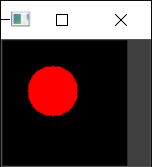# C++ opencv實現幾何圖形繪制

## 1.矩形 rectangle（）

```void rectangle(InputOutputArray img, Rect rec,
const Scalar& color, int thickness = 1,
int lineType = LINE_8, int shift = 0);

img 名稱
rec pt1矩形的頂點 pt2與pt1相對的矩形頂點
color 顏色  也可以用像素存放類Scalar
thickness 寬度 如果是-1，就代表對改矩形進行填充
lineType  類型
shift 移位點坐標中的小數位數。
```

```int main()
{
Mat img = Mat::ones(240, 240, CV_8UC3);
rectangle(img, Rect(20, 20, 100, 100), Scalar(0, 0, 255),7);
imshow("www", img);
waitKey(0);
}
```## 2. 圓 circle（）

```void circle(InputOutputArray img, Point center, int radius,
const Scalar& color, int thickness = 1,
int lineType = LINE_8, int shift = 0);

img 名稱
center 圓心坐標
color 圓環顏色
thickness 正數，則表示圓輪廓的厚度 負數 對該圓填充顏色
lineType  類型
shift  移位中心坐標和半徑值的小數位數。
```

```int main()
{
Mat img1=Mat::zeros(100, 100, CV_8UC3);
circle(img1, Point(40, 40), 20, Scalar(0, 0, 255),-1);//-1 填充
imshow("www", img1);
waitKey(0);
}
```## 3.橢圓 elliple（）

```void ellipse(InputOutputArray img, Point center, Size axes,
double angle, double startAngle, double endAngle,
const Scalar& color, int thickness = 1,
int lineType = LINE_8, int shift = 0);

img 名稱
center 橢圓的中心。
axes 軸 橢圓主軸大小的一半。
angle	橢圓旋轉角度。
startAngle	橢圓弧的起始角，以度表示。
endAngle	橢圓弧的結束角，以度數表示。
color	橢圓顏色。
thickness 正數 橢圓圓弧輪廓的厚度  負數 對橢圓進行填充。
linetype 橢圓邊界類型。
shift 中心坐標和坐標軸值的小數位數。
```

```int main()
{
Mat img1 = Mat::zeros(300, 300, CV_8UC3);
ellipse(img1, Point(100, 100), Size(40, 25), 0, 0, 360, Scalar(0, 0, 255),5);
imshow("111", img1);
waitKey(0);
}
```